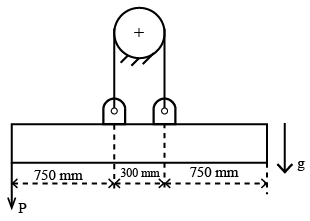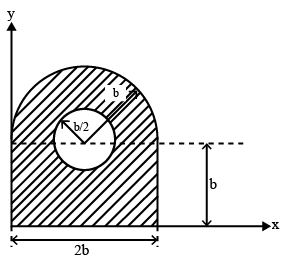# ISRO Scientist or Engineer Refrigeration & Air-Conditioning 2020

Instructions

For the following questions answer them individually

Question 1

# A furnace wall made of steel plate 10 mmthick and thermal conductivity 15 keal/m-hr-$$^\circ C$$ is lined inside with silica brick of 150 mm thick of thermal conductivity 1.75 kcal/m-hr-$$^\circ C$$ and on with outside magnesia bricks of 200 mm thick with thermal conductivity of 4.5 kcal/m-hr-$$^\circ C$$. The total resistance of the composite wall will beQuestion 2

# A water turbine delivering 10 MW power is to be tested with the help of a geometrically similar 1:10 model which runs at the same speed and efficiency. The power developedby the model will beQuestion 3

# A hot fluid is flowing through a long pipe of 4 cm outer diameter and covered with 2 cm thick insulation. It is proposed to reduce the conduction heat loss to the surroundings to one third of the present rate by increasing the same insulation thickness. The additional thickness of insulation required will beQuestion 4

# A uniform beam of mass density 74 kg/m and length 1.8 m is suspended symmetrically as shown in Fig. Calculate the maximum force P that can be supported without tipping the beam. Take the coefficient of static friction between the cord and the cylinder to be 7/22Question 5

# For which of the following situations, zeroth law of thermodynamics will not be applicable?Question 6

# A system undergoes a change of state during which 100 kJ of heat is transferred to it and it does 50 kJ of work. The system is brought back to its original state through a Process during which 120 kJ of heat is transferred to it. The work done by the system in kJ isQuestion 7

# 90 kilograms of ice at $$0^\circ C$$ is completely melted. Find the entropy change in kJ/K, if final temperature is $$0^\circ C$$Question 8

# For a heat engine operating on Carnot cycle, the work output is $$\frac{1}{4}^{th}$$ of the heat transferred to the cold system. The efficiency of the Engine isQuestion 9

# Ideal Diesel cycle consists ofQuestion 10

# Determine the centroid $$(x_c, y_c)$$ of the shaded area as shown in Figure with respect to given coordinate axesOR